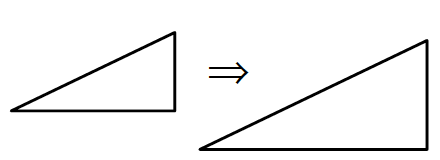### Home > MC2 > Chapter 4 > Lesson 4.3.4 > Problem4-109

4-109.

For each expression below:

• Sketch and label a pair of similar shapes (like those at right or in problem 4-94) that would result in each calculation.

• Rewrite the expression so that the operation is multiplication.

• Calculate the value of the expression.1. $6\div\frac{1}{2}$

Remember. when you divide by a fraction you can also multiply by its reciprocal.

$6·2=?$

$12$

1. $4\div\frac{2}{3}$

$4\left(\frac{3}{2}\right)=?$